## CAT 2019 LRDI Solution Slot 1

Q.1 to 4

A supermarket has to place 12 items (coded A to L) in shelves numbered 1 to 16. Five of these items are types of biscuits, three are types of candies and the rest are types of savouries. Only one item can be kept in a shelf. Items are to be placed such that all items of same type are clustered together with no empty shelf between items of the same type and at least one empty shelf between two different types of items. At most two empty shelves can have consecutive numbers.

The following additional facts are known.

1. A and B are to be placed in consecutively numbered shelves in increasing order.
2. I and J are to be placed in consecutively numbered shelves both higher numbered than the shelves in which A and B are kept.
3. D, E and F are savouries and are to be placed in consecutively numbered shelves in increasing order after all the biscuits and candies.
4. K is to be placed in shelf number 16.
5. L and J are items of the same type, while H is an item of a different type.
6. C is a candy and is to be placed in a shelf preceded by two empty shelves.
7. L is to be placed in a shelf preceded by exactly one empty shelf.

Q.1)In how many different ways  can the items be arranged on the shelves ?

a)1

b)4

c)2

d)8

[bg_collapse view=”button-blue” color=”#ffffff” expand_text=”Correct Answer” collapse_text=”Show Less” ]d)8[/bg_collapse] [bg_collapse view=”button-orange” color=”#4a4949″ expand_text=”View Solution” collapse_text=”Show Less” ]

Solution of Set 1

 1 2 3 4 5 6 7 8 9 10 11 12 13 14 15 16

• We have to arrange 3 types of item (B, C and S) (total 12 items) in 16 shelves space. We can have 1 or 2 empty selves (E) between 2 items.
• It is known that K is on 16th shelves so we put that on 16th ( from condition 4)
• ( From condition 4) It is given that D,E,F will be placed after biscuits and cookies so they will be in last item group. So we will put D,E,F in last shelves in same order and K will be be last in that group.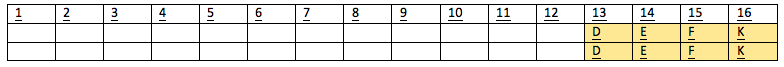• (From condition 6) There should be 2 empty shelve before C.  We also know that C is candy and there are 3 candies.
• We can arrange them in 2 different ways.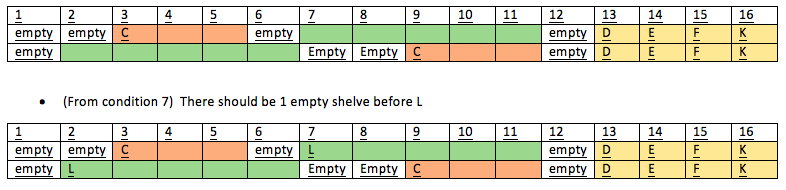• Now (from condition 2), I and J will be placed after A and B . ( A…B….I/J…)
• (From condition 5) L and J are items of the same type
• Mixing above both conditions, we get that I,J,L are of same type and they must be biscuits as they cannot be candies( C is already a candy and they can be only 3 candies in total).
• (From Condition 5) H is an item of a different type than L,J . Thus H will be in cookies group.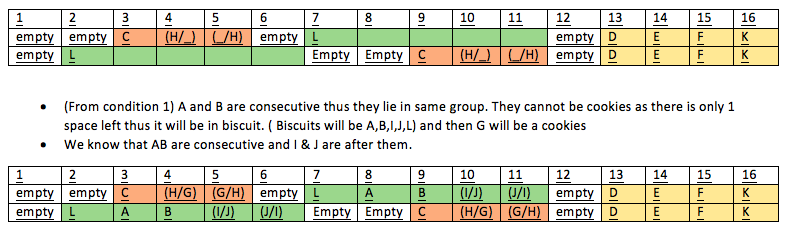[/bg_collapse]Q.2)Which of the following items is not a type of biscuit?

a)G

b)L

c)B

d)A

[bg_collapse view=”button-blue” color=”#ffffff” expand_text=”Correct Answer” collapse_text=”Show Less” ]a)G is not a type of biscuit ( As we can clearly see that it is cookies in both cases)[/bg_collapse] [bg_collapse view=”button-orange” color=”#4a4949″ expand_text=”View Solution” collapse_text=”Show Less” ]

Solution of Set 1

 1 2 3 4 5 6 7 8 9 10 11 12 13 14 15 16

• We have to arrange 3 types of item (B, C and S) (total 12 items) in 16 shelves space. We can have 1 or 2 empty selves (E) between 2 items.
• It is known that K is on 16th shelves so we put that on 16th ( from condition 4)
• ( From condition 4) It is given that D,E,F will be placed after biscuits and cookies so they will be in last item group. So we will put D,E,F in last shelves in same order and K will be be last in that group.• (From condition 6) There should be 2 empty shelve before C.  We also know that C is candy and there are 3 candies.
• We can arrange them in 2 different ways.• Now (from condition 2), I and J will be placed after A and B . ( A…B….I/J…)
• (From condition 5) L and J are items of the same type
• Mixing above both conditions, we get that I,J,L are of same type and they must be biscuits as they cannot be candies( C is already a candy and they can be only 3 candies in total).
• (From Condition 5) H is an item of a different type than L,J . Thus H will be in cookies group.[/bg_collapse]

Q.3)Which of the following can represent the numbers of the empty shelves in a possible arrangement?

a)1,2,6,12

b)1,7,11,12

c)1,5,6,12

d)1,2,8,12

[bg_collapse view=”button-blue” color=”#ffffff” expand_text=”Correct Answer” collapse_text=”Show Less” ]a)1,2,6,12[/bg_collapse] [bg_collapse view=”button-orange” color=”#4a4949″ expand_text=”View Solution” collapse_text=”Show Less” ]

Solution of Set 1

 1 2 3 4 5 6 7 8 9 10 11 12 13 14 15 16

• We have to arrange 3 types of item (B, C and S) (total 12 items) in 16 shelves space. We can have 1 or 2 empty selves (E) between 2 items.
• It is known that K is on 16th shelves so we put that on 16th ( from condition 4)
• ( From condition 4) It is given that D,E,F will be placed after biscuits and cookies so they will be in last item group. So we will put D,E,F in last shelves in same order and K will be be last in that group.• (From condition 6) There should be 2 empty shelve before C.  We also know that C is candy and there are 3 candies.
• We can arrange them in 2 different ways.• Now (from condition 2), I and J will be placed after A and B . ( A…B….I/J…)
• (From condition 5) L and J are items of the same type
• Mixing above both conditions, we get that I,J,L are of same type and they must be biscuits as they cannot be candies( C is already a candy and they can be only 3 candies in total).
• (From Condition 5) H is an item of a different type than L,J . Thus H will be in cookies group.[/bg_collapse]

Q.4)Which of the following statements is necessarily true?

a)There are two empty shelves between the biscuits and the candies.

b)All biscuits are kept before candies.

c)There are at least four shelves between items B and C.

d)All candies are kept before biscuits.

[bg_collapse view=”button-blue” color=”#ffffff” expand_text=”Correct Answer” collapse_text=”Show Less” ]c)There are at least four shelves between items B and C.[/bg_collapse] [bg_collapse view=”button-orange” color=”#4a4949″ expand_text=”View Solution” collapse_text=”Show Less” ]

Solution of Set 1

 1 2 3 4 5 6 7 8 9 10 11 12 13 14 15 16

• We have to arrange 3 types of item (B, C and S) (total 12 items) in 16 shelves space. We can have 1 or 2 empty selves (E) between 2 items.
• It is known that K is on 16th shelves so we put that on 16th ( from condition 4)
• ( From condition 4) It is given that D,E,F will be placed after biscuits and cookies so they will be in last item group. So we will put D,E,F in last shelves in same order and K will be be last in that group.• (From condition 6) There should be 2 empty shelve before C.  We also know that C is candy and there are 3 candies.
• We can arrange them in 2 different ways.• Now (from condition 2), I and J will be placed after A and B . ( A…B….I/J…)
• (From condition 5) L and J are items of the same type
• Mixing above both conditions, we get that I,J,L are of same type and they must be biscuits as they cannot be candies( C is already a candy and they can be only 3 candies in total).
• (From Condition 5) H is an item of a different type than L,J . Thus H will be in cookies group.[/bg_collapse]

Q.5 to 8

Six players – Tanzi, Umeza, Wangdu, Xyla, Yonita and Zeneca competed in an archery tournament. The tournament had three compulsory rounds, Rounds 1 to 3. In each round every player shot an arrow at a target. Hitting the centre of the target (called bull’s eye) fetched the highest score of 5. The only other possible scores that a player could achieve were 4, 3, 2 and 1. Every bull’s eye score in the first three rounds gave a player one additional chance to shoot in the bonus rounds, Rounds 4 to 6. The possible scores in Rounds 4 to 6 were identical to the first three.

A player’s total score in the tournament was the sum of his/her scores in all rounds played by him/her. The table below presents partial information on points scored by the players after completion of the tournament. In the table, NP means that the player did not participate in that round, while a hyphen means that the player participated in that round and the score information is missing.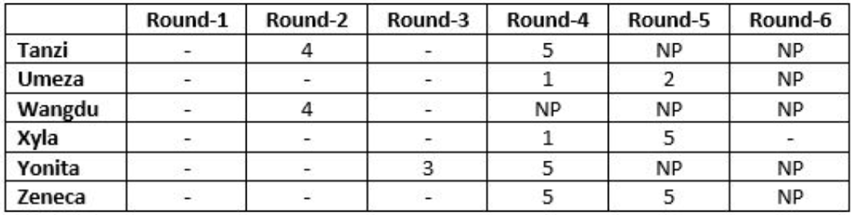The following facts are also known.

1.Tanzi, Umeza and Yonita had the same total score.
2.Total scores for all players, except one, were in multiples of three.
3.The highest total score was one more than double of the lowest total score.
4.The number of players hitting bull’s eye in Round 2 was double of that in Round 3.
5.Tanzi and Zeneca had the same score in Round 1 but different scores in Round 3.

Q.5)What was the highest total score?

a)25

b)21

c)24

d)23

[bg_collapse view=”button-blue” color=”#ffffff” expand_text=”Correct Answer” collapse_text=”Show Less” ]a)25[/bg_collapse] [bg_collapse view=”button-orange” color=”#4a4949″ expand_text=”View Solution” collapse_text=”Show Less” ]of its nostalgic association with a pre-industrial past.

 Round 1 Round 2 Round 3 Round 4 Round 5 Round 6 Total Tanzi – 4 – 5 NP NP Umeza – – – 1 2 NP Wangdu – 4 – NP NP NP Xyla – – – 1 5 – Yonita – – 3 5 NP NP Zeneca – – – 5 5 NP

In this, First thing that we can conclude is that those who played 1 round out of round 4, 5 and 6 must have scored one 5 in their first 3 shots. Similarly 2 and 3 can be concluded.

By this, Xyla must have scored 5 in each round. Tanzi scored a 5 in either round 1 or 3. Umeza must have scored 2 5’s in 3 rounds. And so on.

Accordingly, we can also put a bracket of possible scores for each of them.

For example, Tanzi scored a 4 in round 2 and 5 in round 5 and also a 5 in either round 1 or 3. Hence Tanzi’s total score out of these 3 round can be 14. In the remaining round, Tanzi could have scored 1-4 (not 5 because then round 5 would also be there).

 Round 1 Round 2 Round 3 Round 4 Round 5 Round 6 Total Tanzi – 4 – 5 NP NP 15-18 Umeza – – – 1 2 NP 14-17 Wangdu – 4 – NP NP NP 6-12 Xyla 5 5 5 1 5 – 22-26 Yonita – – 3 5 NP NP 14-17 Zeneca – – – 5 5 NP 21-24

By point 1, Tanzi, Umeza and Yonita had same score. So possible scores for these 3 would be 15-17.

By point 2, only 1 player had scored a non 3x score. Combining this with point 1, we see that possible scores for the can only be 15 or else there will be 3 people with non 3x score.

By point 3, Highest is one more than double of lowest. As we see that highest possible score is 22-26, lowest can only be 11-12. If 11, highest would be 23 and if 12, highest would be 25.

As only one of these could have had a non 3x score, we can eliminate 11 and 23.

So Possible scores now are

 Round 1 Round 2 Round 3 Round 4 Round 5 Round 6 Total Tanzi – 4 – 5 NP NP 15 Umeza – – – 1 2 NP 15 Wangdu – 4 – NP NP NP 12 Xyla 5 5 5 1 5 – 25 Yonita – – 3 5 NP NP 15 Zeneca – – – 5 5 NP 21/24

We can fill some of the scores as per total, in each round.

 Round 1 Round 2 Round 3 Round 4 Round 5 Round 6 Total Tanzi – 4 – 5 NP NP 15 Umeza – – – 1 2 NP 15 Wangdu 4 4 4 NP NP NP 12 Xyla 5 5 5 1 5 4 25 Yonita – – 3 5 NP NP 15 Zeneca – – – 5 5 NP 21/24

Further possible scores for them in first 3 round would be

Tanzi: 5/1, Umeza: 5/5/2, Yonita: 5/2 and Zeneca: 5/5/(1/4)

By point 4, 5 in round 1 are double than round 3.

Now if there is only 1 bullseye in round 3. Umeza and Zeneca doesn’t score 5 in round 3, then they must score 5 in round 2. This will make 5’s in round 2 more than 2. So the case is invalid.

That means there must be 4 bullseyes in round 2.

 Round 1 Round 2 Round 3 Round 4 Round 5 Round 6 Total Tanzi – 4 – 5 NP NP 15 Umeza – 5 – 1 2 NP 15 Wangdu 4 4 4 NP NP NP 12 Xyla 5 5 5 1 5 4 25 Yonita 2 5 3 5 NP NP 15 Zeneca – 5 – 5 5 NP 21/24

By point 5, Tanzi and Zeneca had same score in round 1 but different in round 3.

So one of them must have scored a 5 in either round 1 or 3. This means there are 2 bullseye in round 3 and Umeza must have scored it in round 1 only.

Concluding from this,

 Round 1 Round 2 Round 3 Round 4 Round 5 Round 6 Total Tanzi 5 4 1 5 NP NP 15 Umeza 5 5 2 1 2 NP 15 Wangdu 4 4 4 NP NP NP 12 Xyla 5 5 5 1 5 4 25 Yonita 2 5 3 5 NP NP 15 Zeneca 4 5 5 5 5 NP 24

[/bg_collapse]Q.6)What was Zeneca’s total score?

a)23

b)22

c)24

d)21

[bg_collapse view=”button-blue” color=”#ffffff” expand_text=”Correct Answer” collapse_text=”Show Less” ]c)24[/bg_collapse] [bg_collapse view=”button-orange” color=”#4a4949″ expand_text=”View Solution” collapse_text=”Show Less” ]of its nostalgic association with a pre-industrial past.

 Round 1 Round 2 Round 3 Round 4 Round 5 Round 6 Total Tanzi – 4 – 5 NP NP Umeza – – – 1 2 NP Wangdu – 4 – NP NP NP Xyla – – – 1 5 – Yonita – – 3 5 NP NP Zeneca – – – 5 5 NP

In this, First thing that we can conclude is that those who played 1 round out of round 4, 5 and 6 must have scored one 5 in their first 3 shots. Similarly 2 and 3 can be concluded.

By this, Xyla must have scored 5 in each round. Tanzi scored a 5 in either round 1 or 3. Umeza must have scored 2 5’s in 3 rounds. And so on.

Accordingly, we can also put a bracket of possible scores for each of them.

For example, Tanzi scored a 4 in round 2 and 5 in round 5 and also a 5 in either round 1 or 3. Hence Tanzi’s total score out of these 3 round can be 14. In the remaining round, Tanzi could have scored 1-4 (not 5 because then round 5 would also be there).

 Round 1 Round 2 Round 3 Round 4 Round 5 Round 6 Total Tanzi – 4 – 5 NP NP 15-18 Umeza – – – 1 2 NP 14-17 Wangdu – 4 – NP NP NP 6-12 Xyla 5 5 5 1 5 – 22-26 Yonita – – 3 5 NP NP 14-17 Zeneca – – – 5 5 NP 21-24

By point 1, Tanzi, Umeza and Yonita had same score. So possible scores for these 3 would be 15-17.

By point 2, only 1 player had scored a non 3x score. Combining this with point 1, we see that possible scores for the can only be 15 or else there will be 3 people with non 3x score.

By point 3, Highest is one more than double of lowest. As we see that highest possible score is 22-26, lowest can only be 11-12. If 11, highest would be 23 and if 12, highest would be 25.

As only one of these could have had a non 3x score, we can eliminate 11 and 23.

So Possible scores now are

 Round 1 Round 2 Round 3 Round 4 Round 5 Round 6 Total Tanzi – 4 – 5 NP NP 15 Umeza – – – 1 2 NP 15 Wangdu – 4 – NP NP NP 12 Xyla 5 5 5 1 5 – 25 Yonita – – 3 5 NP NP 15 Zeneca – – – 5 5 NP 21/24

We can fill some of the scores as per total, in each round.

 Round 1 Round 2 Round 3 Round 4 Round 5 Round 6 Total Tanzi – 4 – 5 NP NP 15 Umeza – – – 1 2 NP 15 Wangdu 4 4 4 NP NP NP 12 Xyla 5 5 5 1 5 4 25 Yonita – – 3 5 NP NP 15 Zeneca – – – 5 5 NP 21/24

Further possible scores for them in first 3 round would be

Tanzi: 5/1, Umeza: 5/5/2, Yonita: 5/2 and Zeneca: 5/5/(1/4)

By point 4, 5 in round 1 are double than round 3.

Now if there is only 1 bullseye in round 3. Umeza and Zeneca doesn’t score 5 in round 3, then they must score 5 in round 2. This will make 5’s in round 2 more than 2. So the case is invalid.

That means there must be 4 bullseyes in round 2.

 Round 1 Round 2 Round 3 Round 4 Round 5 Round 6 Total Tanzi – 4 – 5 NP NP 15 Umeza – 5 – 1 2 NP 15 Wangdu 4 4 4 NP NP NP 12 Xyla 5 5 5 1 5 4 25 Yonita 2 5 3 5 NP NP 15 Zeneca – 5 – 5 5 NP 21/24

By point 5, Tanzi and Zeneca had same score in round 1 but different in round 3.

So one of them must have scored a 5 in either round 1 or 3. This means there are 2 bullseye in round 3 and Umeza must have scored it in round 1 only.

Concluding from this,

 Round 1 Round 2 Round 3 Round 4 Round 5 Round 6 Total Tanzi 5 4 1 5 NP NP 15 Umeza 5 5 2 1 2 NP 15 Wangdu 4 4 4 NP NP NP 12 Xyla 5 5 5 1 5 4 25 Yonita 2 5 3 5 NP NP 15 Zeneca 4 5 5 5 5 NP 24

[/bg_collapse]

Q.7)Which of the following statements is true?

a)Xyla was the highest scorer.

b)Zeneca was the highest scorer.

c)Zeneca’s score was 23.

d)Xyla’s score was 23.

[bg_collapse view=”button-blue” color=”#ffffff” expand_text=”Correct Answer” collapse_text=”Show Less” ]a)Xyla was the highest scorer.[/bg_collapse] [bg_collapse view=”button-orange” color=”#4a4949″ expand_text=”View Solution” collapse_text=”Show Less” ]of its nostalgic association with a pre-industrial past.

 Round 1 Round 2 Round 3 Round 4 Round 5 Round 6 Total Tanzi – 4 – 5 NP NP Umeza – – – 1 2 NP Wangdu – 4 – NP NP NP Xyla – – – 1 5 – Yonita – – 3 5 NP NP Zeneca – – – 5 5 NP

In this, First thing that we can conclude is that those who played 1 round out of round 4, 5 and 6 must have scored one 5 in their first 3 shots. Similarly 2 and 3 can be concluded.

By this, Xyla must have scored 5 in each round. Tanzi scored a 5 in either round 1 or 3. Umeza must have scored 2 5’s in 3 rounds. And so on.

Accordingly, we can also put a bracket of possible scores for each of them.

For example, Tanzi scored a 4 in round 2 and 5 in round 5 and also a 5 in either round 1 or 3. Hence Tanzi’s total score out of these 3 round can be 14. In the remaining round, Tanzi could have scored 1-4 (not 5 because then round 5 would also be there).

 Round 1 Round 2 Round 3 Round 4 Round 5 Round 6 Total Tanzi – 4 – 5 NP NP 15-18 Umeza – – – 1 2 NP 14-17 Wangdu – 4 – NP NP NP 6-12 Xyla 5 5 5 1 5 – 22-26 Yonita – – 3 5 NP NP 14-17 Zeneca – – – 5 5 NP 21-24

By point 1, Tanzi, Umeza and Yonita had same score. So possible scores for these 3 would be 15-17.

By point 2, only 1 player had scored a non 3x score. Combining this with point 1, we see that possible scores for the can only be 15 or else there will be 3 people with non 3x score.

By point 3, Highest is one more than double of lowest. As we see that highest possible score is 22-26, lowest can only be 11-12. If 11, highest would be 23 and if 12, highest would be 25.

As only one of these could have had a non 3x score, we can eliminate 11 and 23.

So Possible scores now are

 Round 1 Round 2 Round 3 Round 4 Round 5 Round 6 Total Tanzi – 4 – 5 NP NP 15 Umeza – – – 1 2 NP 15 Wangdu – 4 – NP NP NP 12 Xyla 5 5 5 1 5 – 25 Yonita – – 3 5 NP NP 15 Zeneca – – – 5 5 NP 21/24

We can fill some of the scores as per total, in each round.

 Round 1 Round 2 Round 3 Round 4 Round 5 Round 6 Total Tanzi – 4 – 5 NP NP 15 Umeza – – – 1 2 NP 15 Wangdu 4 4 4 NP NP NP 12 Xyla 5 5 5 1 5 4 25 Yonita – – 3 5 NP NP 15 Zeneca – – – 5 5 NP 21/24

Further possible scores for them in first 3 round would be

Tanzi: 5/1, Umeza: 5/5/2, Yonita: 5/2 and Zeneca: 5/5/(1/4)

By point 4, 5 in round 1 are double than round 3.

Now if there is only 1 bullseye in round 3. Umeza and Zeneca doesn’t score 5 in round 3, then they must score 5 in round 2. This will make 5’s in round 2 more than 2. So the case is invalid.

That means there must be 4 bullseyes in round 2.

 Round 1 Round 2 Round 3 Round 4 Round 5 Round 6 Total Tanzi – 4 – 5 NP NP 15 Umeza – 5 – 1 2 NP 15 Wangdu 4 4 4 NP NP NP 12 Xyla 5 5 5 1 5 4 25 Yonita 2 5 3 5 NP NP 15 Zeneca – 5 – 5 5 NP 21/24

By point 5, Tanzi and Zeneca had same score in round 1 but different in round 3.

So one of them must have scored a 5 in either round 1 or 3. This means there are 2 bullseye in round 3 and Umeza must have scored it in round 1 only.

Concluding from this,

 Round 1 Round 2 Round 3 Round 4 Round 5 Round 6 Total Tanzi 5 4 1 5 NP NP 15 Umeza 5 5 2 1 2 NP 15 Wangdu 4 4 4 NP NP NP 12 Xyla 5 5 5 1 5 4 25 Yonita 2 5 3 5 NP NP 15 Zeneca 4 5 5 5 5 NP 24

[/bg_collapse]

Q.8)What was Tanzi’s score in Round 3?

a)3

b)4

c)5

d)1

[bg_collapse view=”button-blue” color=”#ffffff” expand_text=”Correct Answer” collapse_text=”Show Less” ]d)1[/bg_collapse] [bg_collapse view=”button-orange” color=”#4a4949″ expand_text=”View Solution” collapse_text=”Show Less” ]of its nostalgic association with a pre-industrial past.

 Round 1 Round 2 Round 3 Round 4 Round 5 Round 6 Total Tanzi – 4 – 5 NP NP Umeza – – – 1 2 NP Wangdu – 4 – NP NP NP Xyla – – – 1 5 – Yonita – – 3 5 NP NP Zeneca – – – 5 5 NP

In this, First thing that we can conclude is that those who played 1 round out of round 4, 5 and 6 must have scored one 5 in their first 3 shots. Similarly 2 and 3 can be concluded.

By this, Xyla must have scored 5 in each round. Tanzi scored a 5 in either round 1 or 3. Umeza must have scored 2 5’s in 3 rounds. And so on.

Accordingly, we can also put a bracket of possible scores for each of them.

For example, Tanzi scored a 4 in round 2 and 5 in round 5 and also a 5 in either round 1 or 3. Hence Tanzi’s total score out of these 3 round can be 14. In the remaining round, Tanzi could have scored 1-4 (not 5 because then round 5 would also be there).

 Round 1 Round 2 Round 3 Round 4 Round 5 Round 6 Total Tanzi – 4 – 5 NP NP 15-18 Umeza – – – 1 2 NP 14-17 Wangdu – 4 – NP NP NP 6-12 Xyla 5 5 5 1 5 – 22-26 Yonita – – 3 5 NP NP 14-17 Zeneca – – – 5 5 NP 21-24

By point 1, Tanzi, Umeza and Yonita had same score. So possible scores for these 3 would be 15-17.

By point 2, only 1 player had scored a non 3x score. Combining this with point 1, we see that possible scores for the can only be 15 or else there will be 3 people with non 3x score.

By point 3, Highest is one more than double of lowest. As we see that highest possible score is 22-26, lowest can only be 11-12. If 11, highest would be 23 and if 12, highest would be 25.

As only one of these could have had a non 3x score, we can eliminate 11 and 23.

So Possible scores now are

 Round 1 Round 2 Round 3 Round 4 Round 5 Round 6 Total Tanzi – 4 – 5 NP NP 15 Umeza – – – 1 2 NP 15 Wangdu – 4 – NP NP NP 12 Xyla 5 5 5 1 5 – 25 Yonita – – 3 5 NP NP 15 Zeneca – – – 5 5 NP 21/24

We can fill some of the scores as per total, in each round.

 Round 1 Round 2 Round 3 Round 4 Round 5 Round 6 Total Tanzi – 4 – 5 NP NP 15 Umeza – – – 1 2 NP 15 Wangdu 4 4 4 NP NP NP 12 Xyla 5 5 5 1 5 4 25 Yonita – – 3 5 NP NP 15 Zeneca – – – 5 5 NP 21/24

Further possible scores for them in first 3 round would be

Tanzi: 5/1, Umeza: 5/5/2, Yonita: 5/2 and Zeneca: 5/5/(1/4)

By point 4, 5 in round 1 are double than round 3.

Now if there is only 1 bullseye in round 3. Umeza and Zeneca doesn’t score 5 in round 3, then they must score 5 in round 2. This will make 5’s in round 2 more than 2. So the case is invalid.

That means there must be 4 bullseyes in round 2.

 Round 1 Round 2 Round 3 Round 4 Round 5 Round 6 Total Tanzi – 4 – 5 NP NP 15 Umeza – 5 – 1 2 NP 15 Wangdu 4 4 4 NP NP NP 12 Xyla 5 5 5 1 5 4 25 Yonita 2 5 3 5 NP NP 15 Zeneca – 5 – 5 5 NP 21/24

By point 5, Tanzi and Zeneca had same score in round 1 but different in round 3.

So one of them must have scored a 5 in either round 1 or 3. This means there are 2 bullseye in round 3 and Umeza must have scored it in round 1 only.

Concluding from this,

 Round 1 Round 2 Round 3 Round 4 Round 5 Round 6 Total Tanzi 5 4 1 5 NP NP 15 Umeza 5 5 2 1 2 NP 15 Wangdu 4 4 4 NP NP NP 12 Xyla 5 5 5 1 5 4 25 Yonita 2 5 3 5 NP NP 15 Zeneca 4 5 5 5 5 NP 24

[/bg_collapse]Q- 9 to 12

The Ministry of Home Affairs is analysing crimes committed by foreigners in different states and union territories (UT) of India. All cases refer to the ones registered against foreigners in 2016.
The number of cases – classified into three categories: IPC crimes, SLL crimes and other crimes – for nine states/UTs are shown in the figure below. These nine belong to the top ten states/UTs in terms of the total number of cases registered. The remaining state (among top ten) is West Bengal, where all the 520 cases registered were SLL crimes.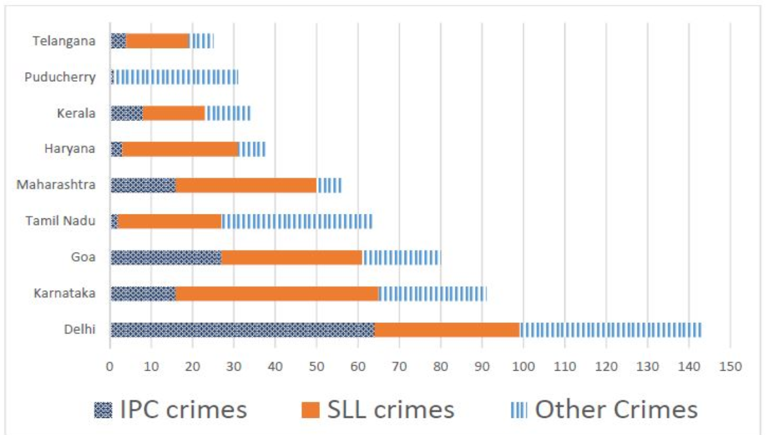The table below shows the ranks of the ten states/UTs mentioned above among ALL states/UTs of India in terms of the number of cases registered in each of the three category of crimes. A state/UT is given rank r for a category of crimes if there are (r‐1) states/UTs having a larger number of cases registered in that category of crimes. For example, if two states have the same number of cases in a category, and exactly three other states/UTs have larger numbers of cases registered in the same category, then both the states are given rank 4 in that category. Missing ranks in the table are denoted by *.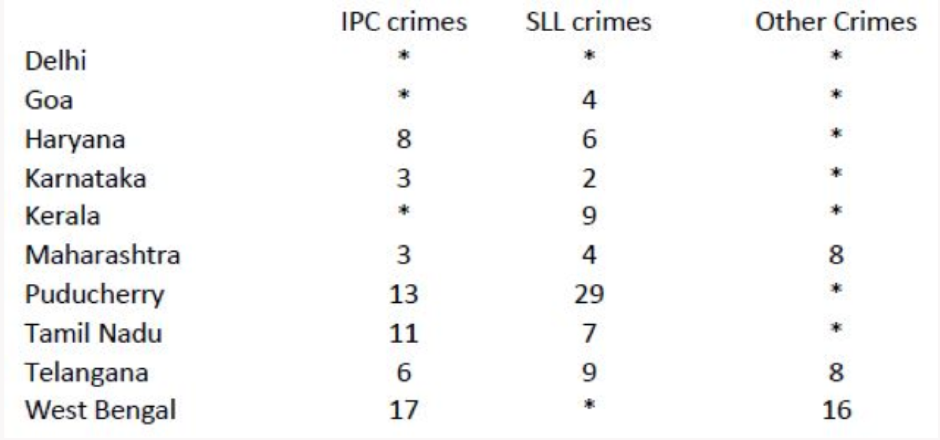Q.9)What is the rank of Kerala in the ‘IPC crimes’ category?

a)5

b)6

[bg_collapse view=”button-blue” color=”#ffffff” expand_text=”Correct Answer” collapse_text=”Show Less” ]a)5[/bg_collapse] [bg_collapse view=”button-orange” color=”#4a4949″ expand_text=”View Solution” collapse_text=”Show Less” ]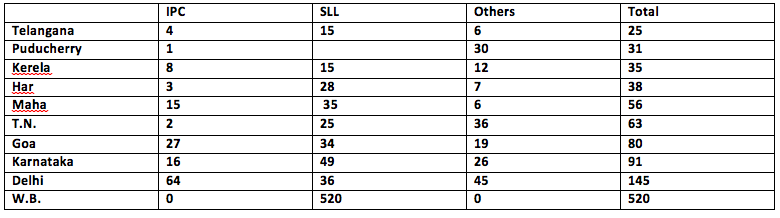[/bg_collapse]

Q.10)In the two states where the highest total number of cases are registered, the ratio of the total number of cases in IPC crimes to the total number in SLL crimes is closest to

a)1:9

b)19:20

c)11:10

d)3:2

[bg_collapse view=”button-blue” color=”#ffffff” expand_text=”Correct Answer” collapse_text=”Show Less” ]1:9[/bg_collapse] [bg_collapse view=”button-orange” color=”#4a4949″ expand_text=”View Solution” collapse_text=”Show Less” ][/bg_collapse]

Q.11)Which of the following is DEFINITELY true about the ranks of states/UT in the ‘other crimes’ category?
ii) Puducherry: 3

a)Neither i) nor ii)

b)Only ii)

c)Only i)

d)Both i) and ii)

[bg_collapse view=”button-blue” color=”#ffffff” expand_text=”Correct Answer” collapse_text=”Show Less” ]d)Both i) and ii)[/bg_collapse] [bg_collapse view=”button-orange” color=”#4a4949″ expand_text=”View Solution” collapse_text=”Show Less” ][/bg_collapse]

Q.12)What is the sum of the ranks of Delhi in the three categories of crimes?

a)5

b)6

[bg_collapse view=”button-blue” color=”#ffffff” expand_text=”Correct Answer” collapse_text=”Show Less” ]a(5)

Delhi’s rank in IPC is 1

Delhi’s rank in SLL  is 3 ( consider West Bengal also as West Bengal is at rank 1)

Delhi’s rank in OTHERS is 1

Sum of ranks = 5[/bg_collapse] [bg_collapse view=”button-orange” color=”#4a4949″ expand_text=”View Solution” collapse_text=”Show Less” ][/bg_collapse]

Q13 to 16

The following table represents addition of two six-digit numbers given in the first and the second rows, while the sum is given in the third row. In the representation, each of the digits 0, 1, 2, 3, 4, 5, 6, 7, 8, 9 has been coded with one letter among A, B, C, D, E, F, G, H, J, K, with distinct letters representing distinct digits.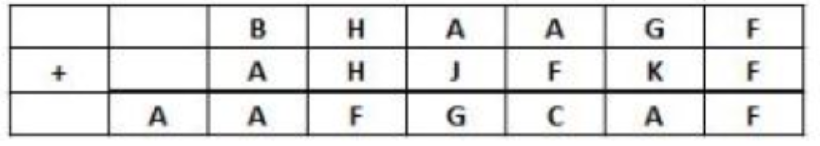Q.13)Which digit does the letter A represent?

[TITA]

[bg_collapse view=”button-blue” color=”#ffffff” expand_text=”Correct Answer” collapse_text=”Show Less” ]1[/bg_collapse] [bg_collapse view=”button-orange” color=”#4a4949″ expand_text=”View Solution” collapse_text=”Show Less” ]

Starting with F, F+F gives us F. only possible F can be 0 here.

 B H A A G 0 A H J 0 K 0 A A 0 G C A 0

In column 5, A+0 gives us C. This is only possible if 1 is carried forward from column 5. This have 2 interpretations, 1) G+K is more than 10, 2) C is one more than A.

Using 2nd and changing C’s into A+1.

In column 3, H+H is equal 0. This can be done if H is 5 or 0. As F is 0, H can be 5 only.

In column 2, B+A is also A. This is possible if B is 0 but F is already 0. Further B can also be 9 for which 1 can be carried forward from column 3.

 9 5 A A G 0 A 5 J 0 K 0 A A 0 G A+1 A 0

As only 1 can be carried forward, A can only be 1.

 9 5 1 1 G 0 1 5 J 0 K 0 1 1 0 G 2 1 0

In column 6, G+K ends up with 1, so G and K can be 6+5 or 7+4 or 8+3 or 9+2. But as 9 and 5 are already done, G and K can be 7/4 or 8/3 only.

In column 4, 1+J is equal to G without any carried forward. Hence, J=G-1. Remaining values for D,E,G,J,K are 3,4,6,7,8. As J=G-1, G can be 4, 7 or 8. Hence, K can be 7, 4 or 3. J can be 3, 6 or 7.[/bg_collapse]Q.14)Which digit does the letter B represent?

a)9

b)8

[bg_collapse view=”button-blue” color=”#ffffff” expand_text=”Correct Answer” collapse_text=”Show Less” ]a)9[/bg_collapse] [bg_collapse view=”button-orange” color=”#4a4949″ expand_text=”View Solution” collapse_text=”Show Less” ]

Starting with F, F+F gives us F. only possible F can be 0 here.

 B H A A G 0 A H J 0 K 0 A A 0 G C A 0

In column 5, A+0 gives us C. This is only possible if 1 is carried forward from column 5. This have 2 interpretations, 1) G+K is more than 10, 2) C is one more than A.

Using 2nd and changing C’s into A+1.

In column 3, H+H is equal 0. This can be done if H is 5 or 0. As F is 0, H can be 5 only.

In column 2, B+A is also A. This is possible if B is 0 but F is already 0. Further B can also be 9 for which 1 can be carried forward from column 3.

 9 5 A A G 0 A 5 J 0 K 0 A A 0 G A+1 A 0

As only 1 can be carried forward, A can only be 1.

 9 5 1 1 G 0 1 5 J 0 K 0 1 1 0 G 2 1 0

In column 6, G+K ends up with 1, so G and K can be 6+5 or 7+4 or 8+3 or 9+2. But as 9 and 5 are already done, G and K can be 7/4 or 8/3 only.

In column 4, 1+J is equal to G without any carried forward. Hence, J=G-1. Remaining values for D,E,G,J,K are 3,4,6,7,8. As J=G-1, G can be 4, 7 or 8. Hence, K can be 7, 4 or 3. J can be 3, 6 or 7.[/bg_collapse]

Q.15) Which among the digits 3, 4, 6 and 7 cannot be represented by the letter D?

[TITA]

[bg_collapse view=”button-blue” color=”#ffffff” expand_text=”Correct Answer” collapse_text=”Show Less” ]7[/bg_collapse] [bg_collapse view=”button-orange” color=”#4a4949″ expand_text=”View Solution” collapse_text=”Show Less” ]

Starting with F, F+F gives us F. only possible F can be 0 here.

 B H A A G 0 A H J 0 K 0 A A 0 G C A 0

In column 5, A+0 gives us C. This is only possible if 1 is carried forward from column 5. This have 2 interpretations, 1) G+K is more than 10, 2) C is one more than A.

Using 2nd and changing C’s into A+1.

In column 3, H+H is equal 0. This can be done if H is 5 or 0. As F is 0, H can be 5 only.

In column 2, B+A is also A. This is possible if B is 0 but F is already 0. Further B can also be 9 for which 1 can be carried forward from column 3.

 9 5 A A G 0 A 5 J 0 K 0 A A 0 G A+1 A 0

As only 1 can be carried forward, A can only be 1.

 9 5 1 1 G 0 1 5 J 0 K 0 1 1 0 G 2 1 0

In column 6, G+K ends up with 1, so G and K can be 6+5 or 7+4 or 8+3 or 9+2. But as 9 and 5 are already done, G and K can be 7/4 or 8/3 only.

In column 4, 1+J is equal to G without any carried forward. Hence, J=G-1. Remaining values for D,E,G,J,K are 3,4,6,7,8. As J=G-1, G can be 4, 7 or 8. Hence, K can be 7, 4 or 3. J can be 3, 6 or 7.[/bg_collapse]

Q.16)Which among the digits 4, 6, 7 and 8 cannot be represented by the letter G?

[TITA]

[bg_collapse view=”button-blue” color=”#ffffff” expand_text=”Correct Answer” collapse_text=”Show Less” ]6[/bg_collapse] [bg_collapse view=”button-orange” color=”#4a4949″ expand_text=”View Solution” collapse_text=”Show Less” ]

Starting with F, F+F gives us F. only possible F can be 0 here.

 B H A A G 0 A H J 0 K 0 A A 0 G C A 0

In column 5, A+0 gives us C. This is only possible if 1 is carried forward from column 5. This have 2 interpretations, 1) G+K is more than 10, 2) C is one more than A.

Using 2nd and changing C’s into A+1.

In column 3, H+H is equal 0. This can be done if H is 5 or 0. As F is 0, H can be 5 only.

In column 2, B+A is also A. This is possible if B is 0 but F is already 0. Further B can also be 9 for which 1 can be carried forward from column 3.

 9 5 A A G 0 A 5 J 0 K 0 A A 0 G A+1 A 0

As only 1 can be carried forward, A can only be 1.

 9 5 1 1 G 0 1 5 J 0 K 0 1 1 0 G 2 1 0

In column 6, G+K ends up with 1, so G and K can be 6+5 or 7+4 or 8+3 or 9+2. But as 9 and 5 are already done, G and K can be 7/4 or 8/3 only.

In column 4, 1+J is equal to G without any carried forward. Hence, J=G-1. Remaining values for D,E,G,J,K are 3,4,6,7,8. As J=G-1, G can be 4, 7 or 8. Hence, K can be 7, 4 or 3. J can be 3, 6 or 7.[/bg_collapse]

Q.17 to 20

Five vendors are being considered for a service. The evaluation committee evaluated each vendor on six aspects – Cost, Customer Service, Features, Quality, Reach, and Reliability. Each of these evaluations are on a scale of 0 (worst) to 100 (perfect). The evaluation scores on these aspects are shown in the radar chart. For example, Vendor 1 obtains a score of 52 on Reliability, Vendor 2 obtains a score of 45 on Features and Vendor 3 obtains a score of 90 on Cost.Q.17)On which aspect is the median score of the five vendors the least?

a)Quality

b)Reliability

c)Cost

d)Customer Service

[bg_collapse view=”button-blue” color=”#ffffff” expand_text=”Correct Answer” collapse_text=”Show Less” ]d)Customer Service[/bg_collapse] [bg_collapse view=”button-orange” color=”#4a4949″ expand_text=”View Solution” collapse_text=”Show Less” ]

solution

Median scores will be the third score in ascending or descending order for any of the 6 aspects. Checking for above 4 aspects, we get median scores as

Quality =62

Reliability=54

Cost=78

Customer Service=50

Least score is for Customer service.

[/bg_collapse]Q.18)A vendor’s final score is the average of their scores on all six aspects. Which vendor has the highest final score?

a)Vendor 3

b)Vendor 2

c)Vendor 4

d)Vendor 1

[bg_collapse view=”button-blue” color=”#ffffff” expand_text=”Correct Answer” collapse_text=”Show Less” ]a)Vendor 3[/bg_collapse] [bg_collapse view=”button-orange” color=”#4a4949″ expand_text=”View Solution” collapse_text=”Show Less” ]

Solution

 Cost Customer Service Features Reach Quality Reliability Total Vender 1 77 55 40 80 72 52 376 Vender 2 82 42 45 58 69 40 336 Vender 3 90 50 55 62 62 75 394 Vender 4 72 70 90 45 40 26 343

Vender 3 has highest final score.

[/bg_collapse]

Q.19)List of all the vendors who are among the top two scorers on the maximum number of aspects is:

a)Vendor 2 and vendor 5

b)Vendor 1 and vendor 5

c)Vendor 2 and vendor 3 and Vendor 4

d)Vendor 1 and vendor 2

[bg_collapse view=”button-blue” color=”#ffffff” expand_text=”Correct Answer” collapse_text=”Show Less” ]b)Vendor 1 and vendor 5[/bg_collapse] [bg_collapse view=”button-orange” color=”#4a4949″ expand_text=”View Solution” collapse_text=”Show Less” ]

If we see the top 2 venders for each of the 6 aspects we will find our answer

 Top 2 vendors Cost 2,3 Customer Service 4,1 Features 4,5 Reach 1,5 Quality 1,2 Reliability 3,5

Vendor 1 and 5 comes for 3 times. Thus Vendor 1 and vendor 5 is our answer.[/bg_collapse]

Q.20)List of all the vendors who are among the top three vendors on all six aspects is:

a)Vendor 1 and vendor 3

b)Vendor 1

c)Vendor 3

d)None of these

[bg_collapse view=”button-blue” color=”#ffffff” expand_text=”Correct Answer” collapse_text=”Show Less” ]c)Vendor 3[/bg_collapse] [bg_collapse view=”button-orange” color=”#4a4949″ expand_text=”View Solution” collapse_text=”Show Less” ]

We will look for top 3 vendors in all aspects

 Top 3 vendors Cost 2,3,1 Customer Service 4,1,3 Features 4,5,3 Reach 1,5,3 Quality 1,2,3 Reliability 3,5,1

Vendor 3 comes for maximum number of time. Thus vendor 3 will be our answer.

[/bg_collapse]

Q.21 to 24

The figure below shows the street map for a certain region with the street intersections marked from a through l. A person standing at an intersection can see along straight lines to other intersections that are in her line of sight and all other people standing at these intersections. For example, a person standing at intersection g can see all people standing at intersections b, c, e, f, h, and k. In particular, the person standing at intersection g can see the person standing at intersection e irrespective of whether there is a person standing at intersection f.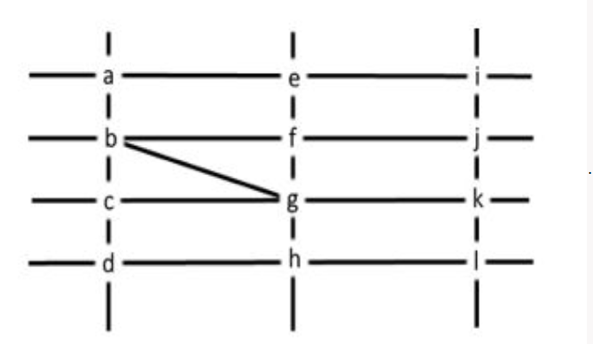Six people U, V, W, X, Y, and Z, are standing at different intersections. No two people are standing at the same intersection.
The following additional facts are known.

1. X, U, and Z are standing at the three corners of a triangle formed by three street segments.
2. X can see only U and Z.
3. Y can see only U and W.
3. U sees V standing in the next intersection behind Z.
4. W cannot see V or Z.
6. No one among the six is standing at intersection d.

Q.21) Who is standing at intersection a?

a)Y

b)No one

c)V

d)W

[bg_collapse view=”button-blue” color=”#ffffff” expand_text=”Correct Answer” collapse_text=”Show Less” ]b)No one[/bg_collapse] [bg_collapse view=”button-orange” color=”#4a4949″ expand_text=”View Solution” collapse_text=”Show Less” ]

Solution

Point 1, Only 2 triangles are possible, BCG and BFG. X, U and Z are standing at these points.

Point 2, there is no one else in straight line of X.

Point 3, Y is on the straight line of U and W.

Point 4, Z and V are standing next to each other while U is also in the same row. Also, Z and are not on BG as then V won’t be standing in the same row. Hence, X is on B or G.

Point 5, W is in different row/column then of V and Z.

Point 6, D is empty.

Case 1: X is at B and BCG is the triangle

 X Z/U U/Z V

As W will be in the column where V and Z are not, W can be in Column 1 or 2. As Column 1 have X, it cannot have W (Point 2). That means Z is below X and U and W in middle Column. Also W is in either Row 1 or 4. By point 3, Y will also be in middle row. But not in front

 w/y X Z U V w/y

Case 2: X is at G and BCG is the triangle

 Z/U Z/U X

Here, D is empty so VZU will be just like the previous case.

 V U Z X

But here, W and Y cannot be in the same row as of U because then one of them will be in column of X. So case invalid.

Case 3: X is at G and BFG is the triangle

 w/y U Z V w/y X

This is also invalid.

Case 4: X is at B and BFG is triangle

 V X Z U

Similar to case 2, this can be negated.

[/bg_collapse]Q.22) Who can V see?

a)U only

b)U and Z only

c)U ,W and Z only

d)Z only

[bg_collapse view=”button-blue” color=”#ffffff” expand_text=”Correct Answer” collapse_text=”Show Less” ]b)U and Z only[/bg_collapse] [bg_collapse view=”button-orange” color=”#4a4949″ expand_text=”View Solution” collapse_text=”Show Less” ]

Solution

Point 1, Only 2 triangles are possible, BCG and BFG. X, U and Z are standing at these points.

Point 2, there is no one else in straight line of X.

Point 3, Y is on the straight line of U and W.

Point 4, Z and V are standing next to each other while U is also in the same row. Also, Z and are not on BG as then V won’t be standing in the same row. Hence, X is on B or G.

Point 5, W is in different row/column then of V and Z.

Point 6, D is empty.

Case 1: X is at B and BCG is the triangle

 X Z/U U/Z V

As W will be in the column where V and Z are not, W can be in Column 1 or 2. As Column 1 have X, it cannot have W (Point 2). That means Z is below X and U and W in middle Column. Also W is in either Row 1 or 4. By point 3, Y will also be in middle row. But not in front

 w/y X Z U V w/y

Case 2: X is at G and BCG is the triangle

 Z/U Z/U X

Here, D is empty so VZU will be just like the previous case.

 V U Z X

But here, W and Y cannot be in the same row as of U because then one of them will be in column of X. So case invalid.

Case 3: X is at G and BFG is the triangle

 w/y U Z V w/y X

This is also invalid.

Case 4: X is at B and BFG is triangle

 V X Z U

Similar to case 2, this can be negated.[/bg_collapse]

Q.23)What is the minimum number of street segments that X must cross to reach Y?

a)2

b)4

c)1

d)3

[bg_collapse view=”button-blue” color=”#ffffff” expand_text=”Correct Answer” collapse_text=”Show Less” ]a)2[/bg_collapse] [bg_collapse view=”button-orange” color=”#4a4949″ expand_text=”View Solution” collapse_text=”Show Less” ]

Solution

Point 1, Only 2 triangles are possible, BCG and BFG. X, U and Z are standing at these points.

Point 2, there is no one else in straight line of X.

Point 3, Y is on the straight line of U and W.

Point 4, Z and V are standing next to each other while U is also in the same row. Also, Z and are not on BG as then V won’t be standing in the same row. Hence, X is on B or G.

Point 5, W is in different row/column then of V and Z.

Point 6, D is empty.

Case 1: X is at B and BCG is the triangle

 X Z/U U/Z V

As W will be in the column where V and Z are not, W can be in Column 1 or 2. As Column 1 have X, it cannot have W (Point 2). That means Z is below X and U and W in middle Column. Also W is in either Row 1 or 4. By point 3, Y will also be in middle row. But not in front

 w/y X Z U V w/y

Case 2: X is at G and BCG is the triangle

 Z/U Z/U X

Here, D is empty so VZU will be just like the previous case.

 V U Z X

But here, W and Y cannot be in the same row as of U because then one of them will be in column of X. So case invalid.

Case 3: X is at G and BFG is the triangle

 w/y U Z V w/y X

This is also invalid.

Case 4: X is at B and BFG is triangle

 V X Z U

Similar to case 2, this can be negated.

[/bg_collapse]Q.24)Should a new person stand at intersection d, who among the six would she see?

a)V and X only

b)U and  W only

c)W and  X only

d)U and  Z only

[bg_collapse view=”button-blue” color=”#ffffff” expand_text=”Correct Answer” collapse_text=”Show Less” ]c)W and  X only[/bg_collapse] [bg_collapse view=”button-orange” color=”#4a4949″ expand_text=”View Solution” collapse_text=”Show Less” ]

Solution

Point 1, Only 2 triangles are possible, BCG and BFG. X, U and Z are standing at these points.

Point 2, there is no one else in straight line of X.

Point 3, Y is on the straight line of U and W.

Point 4, Z and V are standing next to each other while U is also in the same row. Also, Z and are not on BG as then V won’t be standing in the same row. Hence, X is on B or G.

Point 5, W is in different row/column then of V and Z.

Point 6, D is empty.

Case 1: X is at B and BCG is the triangle

 X Z/U U/Z V

As W will be in the column where V and Z are not, W can be in Column 1 or 2. As Column 1 have X, it cannot have W (Point 2). That means Z is below X and U and W in middle Column. Also W is in either Row 1 or 4. By point 3, Y will also be in middle row. But not in front

 w/y X Z U V w/y

Case 2: X is at G and BCG is the triangle

 Z/U Z/U X

Here, D is empty so VZU will be just like the previous case.

 V U Z X

But here, W and Y cannot be in the same row as of U because then one of them will be in column of X. So case invalid.

Case 3: X is at G and BFG is the triangle

 w/y U Z V w/y X

This is also invalid.

Case 4: X is at B and BFG is triangle

 V X Z U

Similar to case 2, this can be negated.[/bg_collapse]

Q.25 to 28

Princess, Queen, Rani and Samragni were the four finalists in a dance competition. Ashman, Badal, Gagan and Dyu were the four music composers who individually assigned items to the dancers. Each dancer had to individually perform in two dance items assigned by the different composers. The first items performed by the four dancers were all assigned by different music composers. No dancer performed her second item before the performance of the first item by any other dancers. The dancers performed their second items in the same sequence of their performance of their first items.

The following additional facts are known.

i) No composer who assigned item to Princess, assigned any item to Queen.
ii) No composer who assigned item to Rani, assigned any item to Samragni.
iii) The first performance was by Princess; this item was assigned by Badal.
iv) The last performance was by Rani; this item was assigned by Gagan.
v) The items assigned by Ashman were performed consecutively. The number of performances between items assigned by each of the remaining composers was the same.

Q.25)Which of the following is true?

a)The third performance was composed by Ashman.

b)The second performance was composed by Gagan.

c)The second performance was composed by Dyu

d)The third performance was composed by Dyu.

[bg_collapse view=”button-blue” color=”#ffffff” expand_text=”Correct Answer” collapse_text=”Show Less” ]c)The second performance was composed by Dyu[/bg_collapse] [bg_collapse view=”button-orange” color=”#4a4949″ expand_text=”View Solution” collapse_text=”Show Less” ]

Solution

• We will make a table with composers on the vertical axis and dancers on the horizontal axis.
• Keep in mind that – Composers can assign 1st, 2nd , 3rd and 4th position in first round and remaining 5th to 8th position in second round.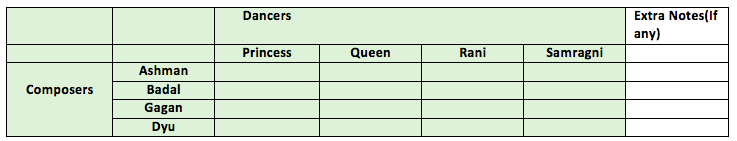• From condition 3, the first performer was by Princess and this item was assigned by Badal. So we assign 1 in that position
• Similarly from condition 4, The last performance was by Rani; this item was assigned by Gagan. So we assign 8 in that position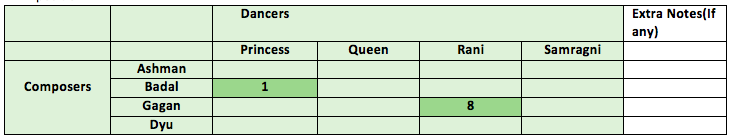• From condition 1, Composer who assigned to Princess did not assigned any item to Queen.
• Similarly, from condition 2, Composer who assigned to Rani did not assigned any item to Samragni.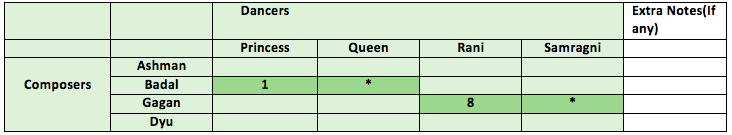• It is given in question that the dancers performed their second items in the same sequence of their performance of their first items. This means that if someone performed at 1st position, he would again perform at 5th Similarly, someone who performs at 3rd position would perform at 7th position.
• Thus princess has performed at 1st position so she would again perform at 5th Similarly, Rani has performed at 8th position, so she would perform at 4th position.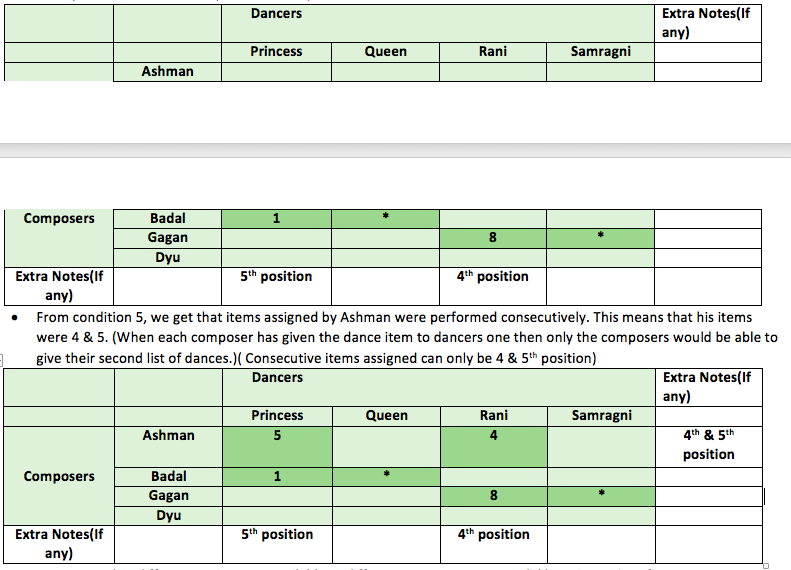• From condition 5, we get that items assigned by Ashman were performed consecutively. This means that his items were 4 & 5. (When each composer has given the dance item to dancers one then only the composers would be able to give their second list of dances.)( Consecutive items assigned can only be 4 & 5th position)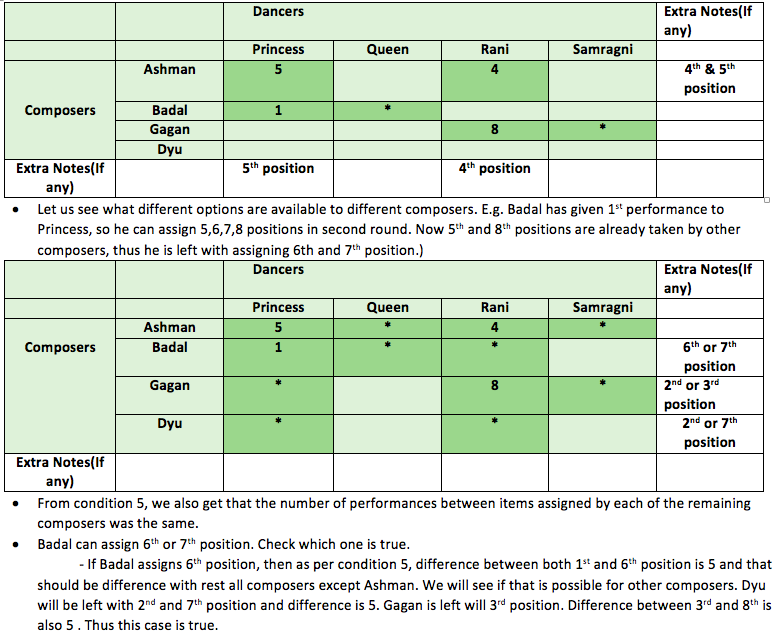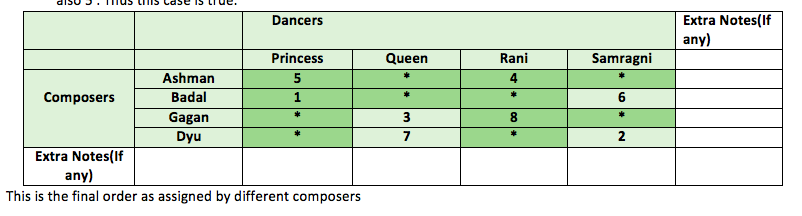[/bg_collapse]Q.26)Which of the following is FALSE?

a)Queen did not perform in any item composed by Gagan.

b)Rani did not perform in any item composed by Badal.

c)Princess did not perform in any item composed by Dyu

d)Samragni did not perform in any item composed by Ashman

[bg_collapse view=”button-blue” color=”#ffffff” expand_text=”Correct Answer” collapse_text=”Show Less” ]a)Queen did not perform in any item composed by Gagan.[/bg_collapse] [bg_collapse view=”button-orange” color=”#4a4949″ expand_text=”View Solution” collapse_text=”Show Less” ]

Solution

• We will make a table with composers on the vertical axis and dancers on the horizontal axis.
• Keep in mind that – Composers can assign 1st, 2nd , 3rd and 4th position in first round and remaining 5th to 8th position in second round.• From condition 3, the first performer was by Princess and this item was assigned by Badal. So we assign 1 in that position
• Similarly from condition 4, The last performance was by Rani; this item was assigned by Gagan. So we assign 8 in that position• From condition 1, Composer who assigned to Princess did not assigned any item to Queen.
• Similarly, from condition 2, Composer who assigned to Rani did not assigned any item to Samragni.• It is given in question that the dancers performed their second items in the same sequence of their performance of their first items. This means that if someone performed at 1st position, he would again perform at 5th Similarly, someone who performs at 3rd position would perform at 7th position.
• Thus princess has performed at 1st position so she would again perform at 5th Similarly, Rani has performed at 8th position, so she would perform at 4th position.• From condition 5, we get that items assigned by Ashman were performed consecutively. This means that his items were 4 & 5. (When each composer has given the dance item to dancers one then only the composers would be able to give their second list of dances.)( Consecutive items assigned can only be 4 & 5th position)[bg_collapse view=”button-blue” color=”#ffffff” expand_text=”Correct Answer” collapse_text=”Show Less” ]c)Vendor 3[/bg_collapse] [bg_collapse view=”button-orange” color=”#4a4949″ expand_text=”View Solution” collapse_text=”Show Less” ]This is the final order as assigned by different composers [/bg_collapse]

Q.27)The sixth performance was composed by:

b)Ashman

c)Gagan

d)Dyu

[bg_collapse view=”button-blue” color=”#ffffff” expand_text=”Correct Answer” collapse_text=”Show Less” ]a)Badal[/bg_collapse] [bg_collapse view=”button-orange” color=”#4a4949″ expand_text=”View Solution” collapse_text=”Show Less” ]

Solution

• We will make a table with composers on the vertical axis and dancers on the horizontal axis.
• Keep in mind that – Composers can assign 1st, 2nd , 3rd and 4th position in first round and remaining 5th to 8th position in second round.• From condition 3, the first performer was by Princess and this item was assigned by Badal. So we assign 1 in that position
• Similarly from condition 4, The last performance was by Rani; this item was assigned by Gagan. So we assign 8 in that position• From condition 1, Composer who assigned to Princess did not assigned any item to Queen.
• Similarly, from condition 2, Composer who assigned to Rani did not assigned any item to Samragni.• It is given in question that the dancers performed their second items in the same sequence of their performance of their first items. This means that if someone performed at 1st position, he would again perform at 5th Similarly, someone who performs at 3rd position would perform at 7th position.
• Thus princess has performed at 1st position so she would again perform at 5th Similarly, Rani has performed at 8th position, so she would perform at 4th position.• From condition 5, we get that items assigned by Ashman were performed consecutively. This means that his items were 4 & 5. (When each composer has given the dance item to dancers one then only the composers would be able to give their second list of dances.)( Consecutive items assigned can only be 4 & 5th position)[/bg_collapse]

Q.28) Which pair of performances were composed by the same composer?

a)The third and the seventh

b)The first and the seventh

c)The second and the sixth

d)The first and the sixth

[bg_collapse view=”button-blue” color=”#ffffff” expand_text=”Correct Answer” collapse_text=”Show Less” ]d)The first and the sixth[/bg_collapse] [bg_collapse view=”button-orange” color=”#4a4949″ expand_text=”View Solution” collapse_text=”Show Less” ]

Solution

• We will make a table with composers on the vertical axis and dancers on the horizontal axis.
• Keep in mind that – Composers can assign 1st, 2nd , 3rd and 4th position in first round and remaining 5th to 8th position in second round.• From condition 3, the first performer was by Princess and this item was assigned by Badal. So we assign 1 in that position
• Similarly from condition 4, The last performance was by Rani; this item was assigned by Gagan. So we assign 8 in that position• From condition 1, Composer who assigned to Princess did not assigned any item to Queen.
• Similarly, from condition 2, Composer who assigned to Rani did not assigned any item to Samragni.• It is given in question that the dancers performed their second items in the same sequence of their performance of their first items. This means that if someone performed at 1st position, he would again perform at 5th Similarly, someone who performs at 3rd position would perform at 7th position.
• Thus princess has performed at 1st position so she would again perform at 5th Similarly, Rani has performed at 8th position, so she would perform at 4th position.• From condition 5, we get that items assigned by Ashman were performed consecutively. This means that his items were 4 & 5. (When each composer has given the dance item to dancers one then only the composers would be able to give their second list of dances.)( Consecutive items assigned can only be 4 & 5th position)[/bg_collapse]

Q.29)What is the minimum possible number of different types of prizes?

[TITA]

[bg_collapse view=”button-blue” color=”#ffffff” expand_text=”Correct Answer” collapse_text=”Show Less” ]2[/bg_collapse] [bg_collapse view=”button-orange” color=”#4a4949″ expand_text=”View Solution” collapse_text=”Show Less” ]The number of items atleast doubles and there are total 100 boxes each containing an item. So minimum types can be 2 as 1st prize having 1 item of type A and 2nd having 99 items of type B. [/bg_collapse]

Q.30)What is the maximum possible number of different types of prizes?

[TITA]

[bg_collapse view=”button-blue” color=”#ffffff” expand_text=”Correct Answer” collapse_text=”Show Less” ]6[/bg_collapse] [bg_collapse view=”button-orange” color=”#4a4949″ expand_text=”View Solution” collapse_text=”Show Less” ]

Similarly to last one, 1 item of type A then 2 of type B then 4 of type C then 8 of type D then 16 of type E then 32 of type F will sum upto 63 items. There cannot be type G because that will cross 100 item barrier. Hence 6.

[/bg_collapse]

Q.31)Which of the following is not possible?

a)There are exactly 60 items of type d.

b)There are exactly 75 items of type e.

c)There are exactly 30 items of type b

d)There are exactly 45 items of type c.

[bg_collapse view=”button-blue” color=”#ffffff” expand_text=”Correct Answer” collapse_text=”Show Less” ]d)There are exactly 45 items of type c.[/bg_collapse] [bg_collapse view=”button-orange” color=”#4a4949″ expand_text=”View Solution” collapse_text=”Show Less” ]

There is 1 item of type A.

If there are exactly 30 items of type B then there will be 60 or more type C. If 69 type C, then This is possible.

If there are exactly 45 type C then there must be 2 to 22 type B only summing upto 48 to 68 items. Now Type D must have at least 90 (double of 45) items but it is not possible.

Exactly 60 type D is possible if there is 1 type A, 9 type B and 30 type C.

75 of type E is also possible. [/bg_collapse]

You ask for the type of item in box 45. Instead of being given a direct answer, you are told that there are 31 items of the same type as box 45 in boxes 1 to 44 and 43 items of the same type as box 45 in boxes 46 to 100.

Q.32)What is the maximum possible number of different types of items?

a)5

b)4

c)3

d)6

[bg_collapse view=”button-blue” color=”#ffffff” expand_text=”Correct Answer” collapse_text=”Show Less” ]a)5[/bg_collapse] [bg_collapse view=”button-orange” color=”#4a4949″ expand_text=”View Solution” collapse_text=”Show Less” ]As type A has only 1 item, this can be type B, C, D, E and F. Hence 5.[/bg_collapse]